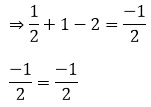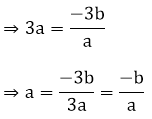×#### Thank you for registering.

One of our academic counsellors will contact you within 1 working day.

Click to Chat

1800-1023-196

+91-120-4616500

CART 0

• 0

MY CART (5)

Use Coupon: CART20 and get 20% off on all online Study Material

ITEM
DETAILS
MRP
DISCOUNT
FINAL PRICE
Total Price: Rs.

There are no items in this cart.
Continue Shopping• Complete JEE Main/Advanced Course and Test Series
• OFFERED PRICE: Rs. 15,900
• View Details

```Chapter 2: Polynomials Exercise – 2.2

Question: 1

Verify that the numbers given alongside of the cubic polynomials below are their zeroes. Also, verify the relationship between the zeroes and coefficients in each of the following cases:

(i) f(x) = 2x3+ x2– 5x + 2; 1/2, 1, – 2

(ii)  g(x) = x3– 4x2 + 5x – 2; 2, 1, 1

Solution:

(i) f(x) = 2x3 + x2 – 5x + 2;  1/2, 1, – 2

(a) By putting x = 1/2 in the above equation, we will get(b) By putting x = 1 in the above equation, we will get

f(1) = 2(1)3 + (1)2 – 5(1) + 2

= 2 + 1 – 5 + 2 = 0

(c) By putting x = -2 in the above equation, we will get

f(−2) = 2(−2)3 + (−2)2 – 5(−2) + 2

= -16 + 4 + 10 + 2 = – 16 + 16 = 0

Now,

Sum of zeroes = α + β + γ = - b/aProduct of the zeroes = αβ + βγ + αγ = c/aHence, verified.

(ii) g(x) = x3 – 4x2 + 5x – 2; 2, 1, 1

(a) By putting x = 2 in the given equation, we will get

g(2) = (2)3 – 4(2)2 + 5(2) – 2

= 8 – 16 + 10 – 2 = 18 – 18 = 0

(b) By putting x = 1 in the given equation, we will get

g(1) = (1)3 – 4(1)2 + 5(1) – 2

= 1 – 4 + 5 – 2

= 0

Now,

Sum of zeroes= α + β + γ =-b/a

⇒ 2 + 1 + 1 = −(−4)

4 = 4

Product of the zeroes = αβ + βγ + αγ = c/a

2 × 1 + 1 × 1 + 1 × 2 = 5

2 + 1 + 2 = 5

5 = 5

αβγ  = –(−2)

2 × 1 × 1 = 2

2 = 2

Hence, verified.

Question: 2

Find a cubic polynomial with the sum, sum of the product of its zeroes is taken two at a time, and product of its zeroes as 3, – 1 and – 3 respectively.

Solution:

Any cubic polynomial is of the form ax3 + bx2 + cx + d:

= x3 – (sum of the zeroes) x2 + (sum of the products of its zeroes) x – (product of the zeroes)

= x3 – 3x2 + (−1)x + (−3)

= k[x3 – 3x2 – x – 3]

k is any non-zero real numbers.

Question: 3

If the zeroes of the polynomial f(x) = 2x3 – 15x2 + 37x – 30, find them.

Solution:

Let, α = a – d, β = a and γ = a + d be the zeroes of the polynomial.

f(x) = 2x3 – 15x2 + 37x – 30And, a (a2 + d2) = 15Question: 4

Find the condition that the zeroes of the polynomial f(x) = x3 + 3px2 + 3qx + r may be in A.P.

Solution:

f(x) = x3 + 3px2 + 3qx + r

Let, a – d, a, a + d be the zeroes of the polynomial.

Then,

The sum of zeroes = – b/a

a + a – d + a + d = -3p 3a = -3p a = -p Since, a is the zero of the polynomial f(x),

Therefore, f(a) = 0

f(a) = a3 + 3pa2 + 3qa + r = 0

Therefore, f(a) = 0f(a) = 0

⇒ a3 + 3pa2 + 3qa + r = 0

= ⇒ (−p)3 + 3p(−p)2 + 3q(−p) + r = 0

= − p3 + 3p3 – pq + r = 0

= 2p3 – pq + r = 0

Question: 5

If zeroes of the polynomial f(x) = ax3 + 3bx2 + 3cx + d are tin A.P., prove that 2b3 – 3abc + a2d = 0.

Solution:

f(x) = x3 + 3px2 + 3qx + r

Let, a – d, a, a + d be the zeroes of the polynomial.

Then,

The sum of zeroes = - b/a

a + a – d + a + d = – 3b/aSince, f(a) = 0

⇒ a(a2) + 3b(a)2 + 3c(a) + d = 0

⇒ a(a2) + 3b(a)2 + 3c(a) + d = 0Question: 6

If the zeroes of the polynomial f(x) = x3 – 12x2 + 39x + k are in A.P., find the value of k.

Solution:

f(x) = x3 – 12x2 + 39x + k

Let, a-d, a, a + d be the zeroes of the polynomial f(x).

The sum of the zeroes = 12

3a = 12

a = 4

Now,

f(a) = 0

f(a) = a3 – 12a2 + 39a + k f(4) = 43 – 12(4)2 + 39(4) + k = 0

f(4) = 43 –12(4)2 + 39(4) + k = 0

64 – 192 + 156 + k = 0

k = – 28
```### Course Features

• 728 Video Lectures
• Revision Notes
• Previous Year Papers
• Mind Map
• Study Planner
• NCERT Solutions
• Discussion Forum
• Test paper with Video Solution